# If Cell is This Value or That Value

IF function is frequently used in Excel worksheet to return you expect “true value” or “false value” based on the result of logical test. If you want to see if a cell is A or B, and if one of them is true, true value is returned and if none of them is true, false value is returned. It seems that we can build a formula with IF function to solve this problem because in this case there are two results “if true value” and “if false value”, and the final result is retrieved from the two results based on the logical test result. But, unlike normal IF formula, there are two logical tests here “cell=A” or “cell=B”, so except IF function, we also use OR function inside IF function to solve this problem. See example below.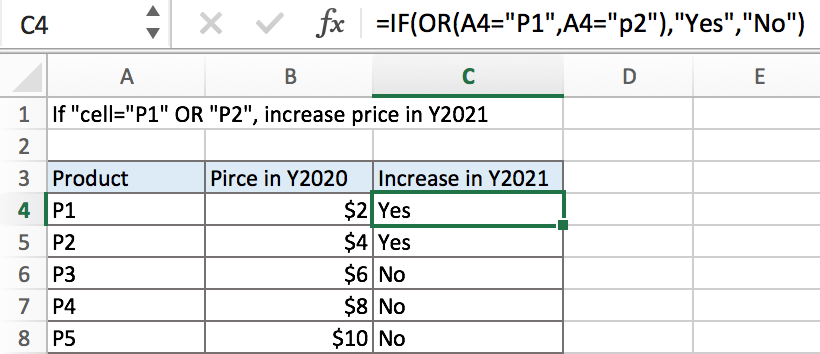In above example, in product list, if cell is “P1” or “P2”, its price will increase in a new year, we will mark “Yes” for them in “Increase in Y2021” list. Otherwise, if cell is not “P1” or “P2”, the price will not increase in Y2021, we will mark “No” in “Increase in Y2021” list. IF function can handle this case effectively, it returns either “Yes” or “No”.

## FORMULA

To test if cell equals a certain text string, the generic formula is:

=IF(OR(A1=”A”,A1=”B”),”true value”,”false value”)

Formula in this example:

=IF(OR(A4=”P1″,A4=”P2″),”Yes”,”No”)

## EXPLANATION

OR function is often used as logical test part inside IF function. It can test multiple logical conditions at one time, and if one of the multiple conditions is true, it returns true. In this example, if A4=”P1” is true, or A4=”P2” is true, OR delivers TRUE to IF function.

Syntax: OR (logical1, [logical2], …)

IF function allows you to create a logical comparison between your value and reference value (for example “A1>0”), and set true value and false value what you expect to return as test results. IF function returns one of the two results based on logical comparison result.

Syntax: IF(logica_test,[value_if_true],[value_if_false])

In this example, we want to mark “Yes” for those cells contain “P1” or “P2”. In normal situation, there is only one logical condition in IF formula, for example IF(cell=”P1”,”Yes”,”No”), but there are two conditions cell=”P1” and cell=”P2”, IF returns true value is one of them is true. As this is a OR relationship, so we add OR function inside IF, and IF logical test is consisted of OR function with the two logical conditions: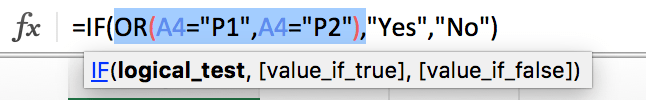x
How to Show All Named Ranges in Excel

To test if A4 is P1 or P2 or not matched, we create the logical comparison OR(A4=”P1″,A4=”P2″):

• The symbol = is a logical operator that means “equal to”
• P1 and P2 are enclosed in double quotes “” as they are texts
• Double quotes “” should not be ignored in this case. If missing double quotes, Excel will prompts warning message that the formula you typed contains an error, if you ignore the error, #VALUE! is displayed in cell

After running the logical test, IF function retrieves true or false result based on the test result. In this example, for the two arguments “value_if_true” and “value_if_false”, we set “Yes” as true result and “No” as false result. So the formula is: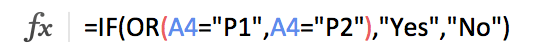In this example, A4 is P1, logical test result is true, so IF function returns True value “Yes”.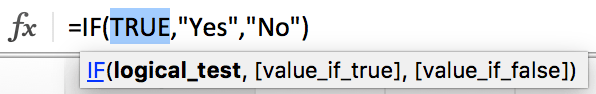For B6 which records P3, it cannot match either of the two conditions, so OR function returns False and deliver false result to IF function, IF retrieves False value “No” from the two results.

In IF, true value and false value can be set as empty string “”, a text, a number or a formula, you can set what you expect to these two values.

## MORE COMPLICATED EXAMPLE

In example below, if product is P1 or P2, price in Y2021 should be increased by 20%. So, if the logical test is true, true value is current price*1.2, as old price is saved in B4, so we set B4*1.2 as true value. If logical test is false, there is no need to update price, so keep original price in B4 as new price, set B4 as false value. In this example, true value is a formula, and false value is a cell reference.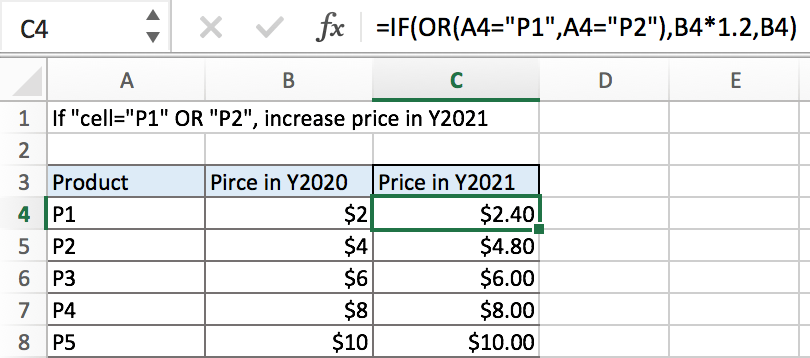### Related Functions

• Excel IF function
The Excel IF function perform a logical test to return one value if the condition is TRUE and return another value if the condition is FALSE. The IF function is a build-in function in Microsoft Excel and it is categorized as a Logical Function.The syntax of the IF function is as below:= IF (condition, [true_value], [false_value])….
• Excel OR Function
The Excel OR function used to test multiple conditions and returns TRUE if any of the conditions are TRUE.The syntax of the OR function is as below:=OR(logical1, [logical2], …)…
Related Posts

Fix #N/A Error For VLOOKUP From Another Sheet

This post will show you how to fix the #N/A error why it occurs when you extract values from another sheet using VLOOKUP function in Excel 2016,2013,2010 or other Excel versions. How can you correct a #N/A error in VLOOKUP ...

How to Average Only Positive or Negative Numbers of a Range

Suppose both positive numbers and negative numbers exist in a table. If we want to know the average of only positive numbers in this table, we can create a formula to get average of all positive numbers with all negative ...

VLOOKUP with Two Lookup Tables

VLOOKUP is one of the key functions among all lookup & reference functions in Excel. Today we will show you the application of VLOOKUP function when there are two lookup tables. EXAMPLE Table1 and table2 record the rates of Y2020 ...

Approximate Match with Multiple Criteria by INDEX & MATCH

In Excel, INDEX function and MATCH function are often used together for returning data from specific position. And MATCH function is one of Excel lookup & reference functions that can return approximate value by setting match type. Above all, through ...

How to Statistic Pass 4 Out of 5 Rounds in a Competition by Excel Functions

Suppose you are counting the numbers of participants who are the winners in a competition. And the passing line is provided as pass 4 out of 5 rounds of games. You need to know if participant pass the game or ...

Average the Last N Numeric Values in Excel

AVERAGE function is one of the most popular functions in Excel. Apply AVERAGE together with some other functions, we can calculate average simply for some complex situations. In this article, we will introduce you to calculate average of the last ...

How to Average and Ignore Errors in Excel

This post will guide you how to average a list of values and ignoring any errors in the given range in Excel 2013/2016 or Excel office 365. You can use the AVERAGEIF function or AGGREGATE function to calculate the average ...

How to Subtotal Values for Groups and Only Keep One Subtotal for A Group in Column

Sometimes we may meet the case that subtotal values for different groups and only record a subtotal value for one group in a column, for other cells in this group, keep them blank. Today we will introduce you how can ...

Running Count of Occurrence List

This post will guide you how to get running count of an occurrence in a list with formula in Excel 2013/2016 or Excel office 365. How to create a running count of certain values that appear in the given range ...

How to Count Unique Numeric Values with Criteria in a Range in Excel

We have talked that how to count unique numeric values in a data set in Excel in the previous post. And this post will guide you how to count unique numeric values with criteria in the range in Excel 2013/2016 ...

Sidebar